# AP Chemistry : Calculating the Equilibrium Concentrations

## Example Questions

### Example Question #1 : Calculating The Equilibrium Concentrations

Consider formation of Nitrogen monoxide: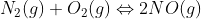with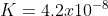.  If the initial concentration of N2 was 0.085M and O2 was 0.038 M, what is the concentration of nitrogen monoxide at equilibrium?

1.1 x 10-4  M

1.2 x 10-6 M

1.2 x 10-5 M

1.1 x 10-3 M

3.2 x 10-8 M

1.2 x 10-5 M

Explanation: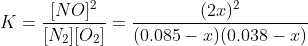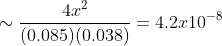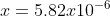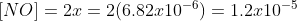### Example Question #661 : Ap Chemistry

Given the ICE table below, what are the signs on the terms that would appear in the “Change” row?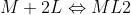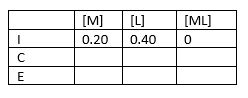-x, -x, +x

+x, +4x, -x

-x, -2x, +x

+x, +2x, +x

+x, +x, +x

-x, -2x, +x

Explanation:

Based on the balanced equation, one would use -x, -2x, and +x.

### Example Question #1 : Calculating The Equilibrium Concentrations

Find the equilibrium concentrations for C in the following chemical reaction:  A + B -> 2C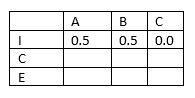K = 9.0 x 10-8

1.5 x 10-4 M

1.1 M

3.0 x 10-3 M

0.12 M

1.2 x 10-2 M

1.5 x 10-4 M

Explanation: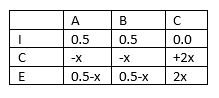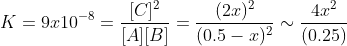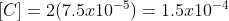### Example Question #1 : Calculating The Equilibrium Concentrations

What is the equilibrium concentration for A- in the following reaction if the starting concentration of HA is 0.60 M?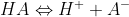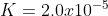1.2 x 10-5 M

2.3 x 10-3 M

3.5 x 10-3 M

1.2 M

2.3 x 10-4 M

3.5 x 10-3 M

Explanation: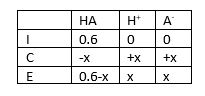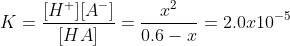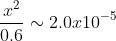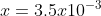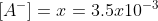### Example Question #1 : Calculating The Equilibrium Concentrations

What is the equilibrium concentration for A- in the following reaction if the starting concentration of HA is 0.30 M?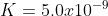3.9 x 10-5 M

1.5 x 10-4 M

2.1 M

2.1 x 10-3 M

1.5 x 10-3 M

3.9 x 10-5 M

Explanation: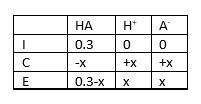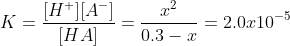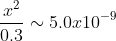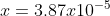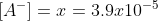### All AP Chemistry Resources